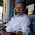### Understanding How to Transpose Without Really Trying: EMII Notes 2014_08_09 Part II

Summary of what's gone on before.  Got through the index notation for gradients and whatnot.  I was left a little bit baffled by the notation for the orthogonal transpose identity.  Consequently, I'm digging back into it.

In this set of notes, the transpose, orthogonal identity, $M_{ki}M_{kj} = \delta_{ij}$, is first hammered out.  It then becomes obvious what's going on.  The dummy summing of the two row indices gave us the equivalent of a matrix multiply where it's row times row instead of row times column.  The rows of a matrix that should have been transposed however are the same as the columns of one that wasn't.  In other words by forcing a different type of matrix multiply, they teased out the transpose for free.

Here's the hammering through bit.

Let's take as an example, the simple rotation matrix about the z axis.  Keep in mind that it has already been explained above why this will work for any orthogonal matrices and that this is just a concrete version that I already happened to work out before figuring out the general case above.

$M_z = \begin{pmatrix} cos \theta & sin \theta & 0\\ -sin \theta & cos \theta & 0\\ 0 & 0 & 1 \end{pmatrix}$

Now, with the multiply defined as above, we will get three resulting matrices for $k=1$, $k=2$, and $k=3$, and then sum them all together.  The k's are fixed, so the only combinations of terms that need to be multiplied are the i's and the j's .  For each k, we'll get the 9 terms indexed by i and j.  So, for the k's, 1 through 3, respectively, we get:

$\begin{pmatrix} cos^2 \theta & cos \theta sin \theta & 0\\ sin \theta cos \theta & sin^2 \theta & 0\\ 0 & 0 & 0 \end{pmatrix}+\begin{pmatrix} sin^2 \theta & -sin \theta cos \theta & 0\\ -cos \theta sin \theta & cos^2 \theta & 0\\ 0 & 0 & 0 \end{pmatrix}+\begin{pmatrix} 0 & 0 & 0\\ 0 & 0 & 0\\ 0 & 0 & 1 \end{pmatrix}$

which adds term by term to give $\delta_{ij}$.

NOTE:  Look at this a lot!  The multiply method of swizzling through all the combinations of i and j tends to slip my mind!Yurlungur said…
Well done! I remember really struggling with this sort of thing when I was first starting on GR.

It's not terribly enlightening, but I often found it helpful to prove index identities to myself using "brute force" by programming the summation into a computer and confirming the left-hand side is the same as the right-hand side. The reason I would do this is sort of two-fold.

Sometimes it was helpful just to prove to myself the identity worked. I didn't really believe it until I saw it in action.

And sometimes I misunderstood the index notation and got the identity wrong! The brute force would make that clear.Hamilton Carter said…
Thanks Yurlunger!

I like your idea about programming the identities. I guess I'll get better with practice, but these are pretty difficult for me to see now, and a computer program, as you pointed out would force me to confront the inconsistencies in my thinking about indices more quickly.

### Cool Math Tricks: Deriving the Divergence, (Del or Nabla) into New (Cylindrical) Coordinate Systems

The following is a pretty lengthy procedure, but converting the divergence, (nabla, del) operator between coordinate systems comes up pretty often. While there are tables for converting between common coordinate systems, there seem to be fewer explanations of the procedure for deriving the conversion, so here goes!

What do we actually want?

To convert the Cartesian nabla

to the nabla for another coordinate system, say… cylindrical coordinates.

What we’ll need:

1. The Cartesian Nabla:

2. A set of equations relating the Cartesian coordinates to cylindrical coordinates:

3. A set of equations relating the Cartesian basis vectors to the basis vectors of the new coordinate system:

How to do it:

Use the chain rule for differentiation to convert the derivatives with respect to the Cartesian variables to derivatives with respect to the cylindrical variables.

The chain rule can be used to convert a differential operator in terms of one variable into a series of differential operators in terms of othe…

### The Valentine's Day Magnetic Monopole

There's an assymetry to the form of the two Maxwell's equations shown in picture 1.  While the divergence of the electric field is proportional to the electric charge density at a given point, the divergence of the magnetic field is equal to zero.  This is typically explained in the following way.  While we know that electrons, the fundamental electric charge carriers exist, evidence seems to indicate that magnetic monopoles, the particles that would carry magnetic 'charge', either don't exist, or, the energies required to create them are so high that they are exceedingly rare.  That doesn't stop us from looking for them though!

Keeping with the theme of Fairbank and his academic progeny over the semester break, today's post is about the discovery of a magnetic monopole candidate event by one of the Fairbank's graduate students, Blas Cabrera.  Cabrera was utilizing a loop type of magnetic monopole detector.  Its operation is in concept very simpl…